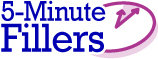#5-Minute Fillers: Math and Time

Volume 39

Roman Numeral Math
Builds math computation skills and Roman numeral knowledge

Provide three math problems that involve adding, subtracting, or multiplying Roman numerals. Create problems appropriate for your grade level. For example, a teacher in grade 5 might provide the following problems:

XII + XXXVI = XLVIII (12 + 36 = 48)
CXXIX - LXI = LXVIII (129 - 61 = 68)
XIV X IV = LVI (14 X 4 = 56)Pose the following question to students to start a lively discussion, or use is as a prompt for a quick journal-writing activity: What if you could be really good at something or have a special skill that you do not have now? What ability would you want to have? Why?

Article by Gary Hopkins
Education World®